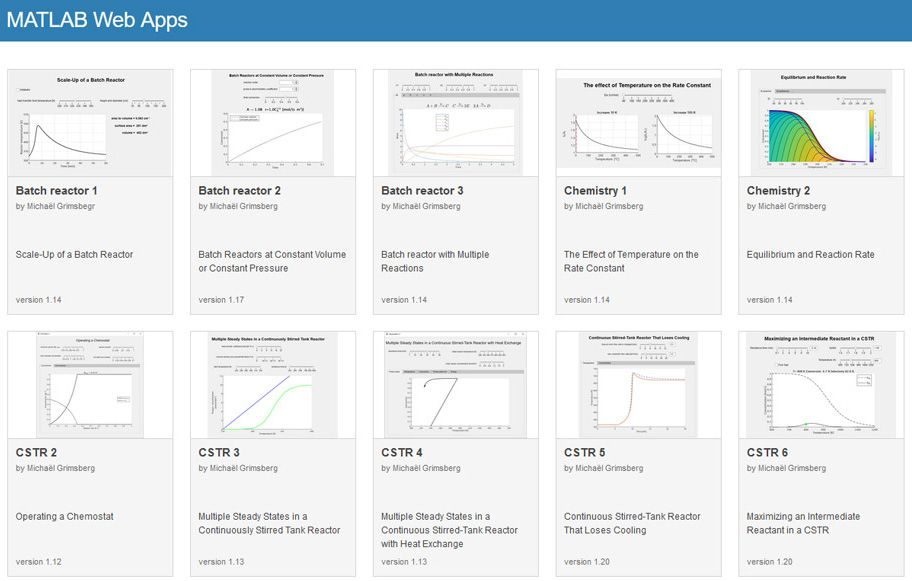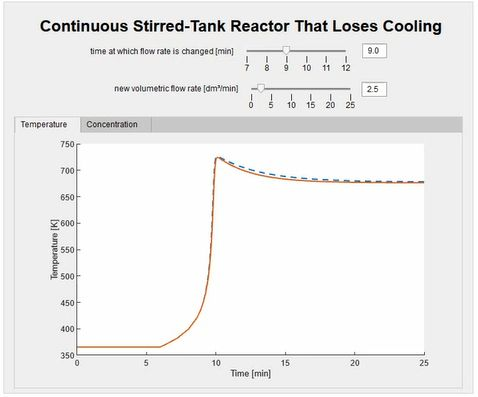# Using Virtual Labs to Teach Reaction Engineering

By Michaël Grimsberg, Lund University

Reaction Engineering introduces third-year chemical engineering students at Lund University to the principles of reactor analysis, sizing, and design. Like other similar courses, it was traditionally taught with a heavy emphasis on theory and the derivation of mathematical equations. Students completed numerous exercises but rarely developed an intuitive understanding of the combined effects of physical processes and chemical reactions that take place in an industrial reactor.

Recognizing this shortcoming, we shifted the focus from mere theory to digital experimentation. I created a set of MATLAB® apps that enable students to interactively modify key reaction parameters and see how their changes affect outcomes (Figure 1). I’ve also begun using MATLAB Grader™ in the course. My teaching assistants (TAs) now spend considerably less time on simple code correction and more time helping students understand the model structure, a particular advantage when the course moved online during the COVID-19 pandemic. Both the apps and MATLAB Grader are integrated into the university's learning management system (LMS).Figure 1. MATLAB web apps used in Reaction Engineering.

## Creating and Hosting MATLAB Apps

The Reaction Engineering apps are designed to get students thinking critically about the effects on reaction performance of processes that take place in batch reactors and continuous stirred-tank reactors (CSTRs). During one class, I ask the students to imagine what would happen if a CSTR cooling system were to malfunction. The students recognize that if no action is taken, the temperature and pressure will rise until the reactor explodes. I then ask, if they could control the reactor’s volumetric flow rate, would they increase or decrease the flow rate to save the reactor?

The answer to this question is not intuitive; most students incorrectly recommend reducing the flow. To show them how increasing the flow would reduce the overall energy in the system, I have them experiment with a MATLAB app that lets them control the flow rate and track the temperature within the reactor over time (Figure 2). The app gives them a much better understanding of the reactor’s energy balance than they could get by completing rote numeric calculations.Figure 2. MATLAB app for visualizing the temperature of a CSTR with a malfunctioning cooling system.

Because Lund University has a Campus-Wide License, students can download the apps I developed and run them on their own computers. However, I decided to make the apps available as interactive web apps via MATLAB Web App Server™. I was then able to provide instructions for assignments onscreen alongside the app. MATLAB Web App Server also made it possible to integrate the apps with Canvas, our LMS, making them easier to access (Figure 3).

When the pandemic forced us to begin teaching online, my colleagues and I had to figure out how to both manage and engage the approximately 100 students in the course. About two weeks before classes were set to begin, I decided to use MATLAB Grader for virtual lab assignments. Starting with the templates for solving differential and nonlinear equations in MATLAB that I previously created for the course, it took me no more than a few days to create and test interactive lab exercises with MATLAB Grader (Figure 4).

MATLAB Grader proved to be an important addition because it provided students with immediate feedback on their work. For example, in the second lab, students simulate a chemical reaction that requires them to solve eight differential equations in MATLAB. Under normal circumstances, 25 to 30 students would be working in a lab with two TAs. Even with that number of students, it takes a lot of time for the TAs to answer questions, which mainly concern simple coding errors, and check each student’s work. With MATLAB Grader, we can automatically check the work of all 100 students and provide real-time feedback. TAs can then give their attention to students who need extra help with their code.

## Student Feedback and Plans for Next Year

While we did not conduct formal course surveys on the use of MATLAB Grader and apps, students commented informally that the apps helped them learn new concepts. They also said they liked the immediate feedback provided by MATLAB Grader.

The changes we made in Reaction Engineering have attracted attention outside Lund University: I’ve been contacted by another university in Sweden that is interested in using MATLAB Grader and MATLAB Web App Server to implement similar enhancements to their own curriculum.

Lund University is among the more than 1500 universities worldwide that provide campus-wide access to MATLAB and Simulink. With a Campus-Wide License, researchers, faculty, and students have access to a common configuration of products, at the latest release level, for use anywhere—in the classroom, at home, in the lab, or in the field.